NCERT Exemplar Class 11 Maths Chapter 1 Sets are part of NCERT Exemplar Class 11 Maths. Here we have given NCERT Exemplar Class 11 Maths Chapter 1 Sets.

## NCERT Exemplar Class 11 Maths Chapter 1 Sets

Q1. Write the following sets in the roaster from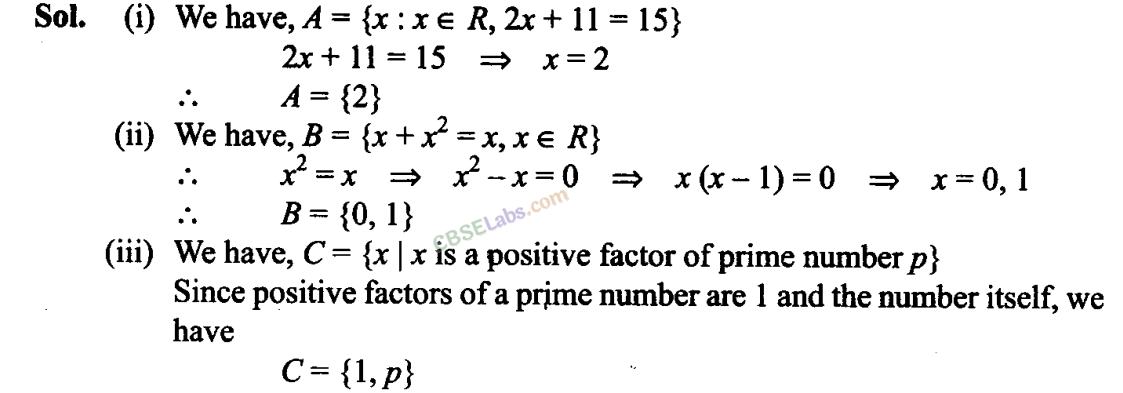Q2. Write the following sets in the roaster form: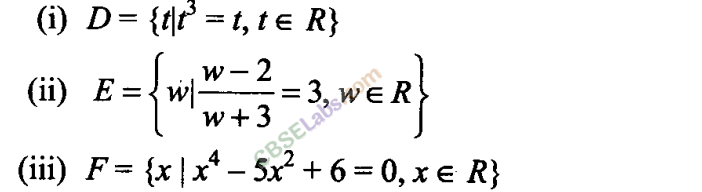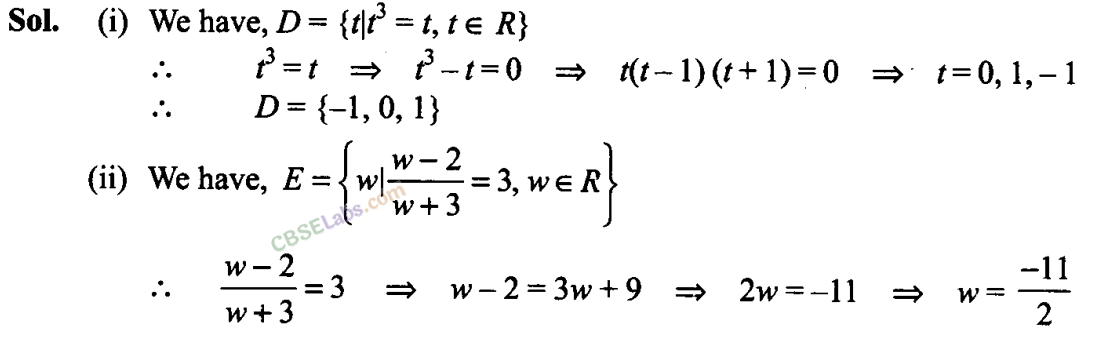Q3. If Y = {x\x is a positive factor of the number 2P(2P – 1), where 2P – 1 is a prime number}. Write Y in the roaster form.

Sol: Y- {x | x is a positive factor of the number 2P-1 (2P – 1), where 2P – 1 is a prime number}.
So, the factors of 2P-1 are 1,2,22,23,…, 2P- 1.
Y= {1,2,22,23, …,2p-1,2p-1}

Q4. State which of the following statements are true and which are false. Justify your answer.
(i) 35 ∈ {x | x has exactly four positive factors}.
(ii) 128 e {y | the sum of all the positive factorsofy is 2y}
(iii) 3∉{x|x4-5x3 + 2jc2-112x + 6 = 0}
(iv) 496 ∉{y | the sum of all the positive factors of y is 2y}.
Sol: (i) The factors of 35 are 1, 5, 7 and 35. So, 35 is an element of the set. Hence, statement is true.

(ii) The factors of 128 hre 1,2,4, 8, 16, 32, 64 and 128.
Sum of factors = 1 + 2 + 4 + 8 + 16 + 32 + 64 + 128 = 255 * 2 x 128 Hence, statement is false.

(iii) We have, x4 – 5x3 + 2x2 – 1 12jc + 6 = 0 Forx = 3, we have
(3)4 – 5(3)3 + 2(3)2 – 112(3) + 6 = 0
=> 81 – 135 + 18-336 + 6 = 0
=>    -346 = 0, which is not true.
So 3 is not an element of the set
Hence, statement is true.

(iv) 496 = 24 x 31
So, the factors of 496 are 1,2,4, 8, 16,31,62, 124,248 and 496.
Sum of factors = 1 +2 + 4 + 8+ 16 + 31+62+124 + 248 + 496 = 992 = 2(496)
So, 496 is the element of the set Hence, statement is false

Q5. Given L, = {1,2, 3,4},M= {3,4, 5, 6} and N= {1,3,5}
Verify that L-(M⋃N) = (L-M)⋂(L-N)
Sol: Given L,= {1,2, 3,4}, M= {3,4,5,6} and N= {1,3,5}
M⋃N= {1,3,4, 5,6}
L – (M⋃N) = {2}
Now, L-M= {1, 2} and L-N= {2,4}
{L-M) ⋂{L-N)= {2}
Hence, L-{M⋃N) = {L-M) ⋂ (L-N).

Q6. If A and B are subsets of the universal set U, then show that
(i) A⊂ A∪ B
(ii) A⊂B⟺A∪B = B
(iii) (A∩B)⊂ AQ7. Given that N= {1,2,3, …, 100}. Then write
(i) the subset of N whose elements are even numbers.
(ii) the subset of N whose element are perfect square numbers.
Sol: We have, N= {1,2, 3,4,…, 100}
(i) subset of N whose elements are even numbers = {2,4, 6, 8,…, 100}
(ii) subset of N whose elements are perfect square = {1,4, 9, 16, 25, 36,49, 64, 81, 100}

Q8. If X= {1, 2, 3}, if n represents any member of X, write the following sets containing all numbers represented by
(i) 4n
(ii) n + 6
(iii) n/2
(iv) n-1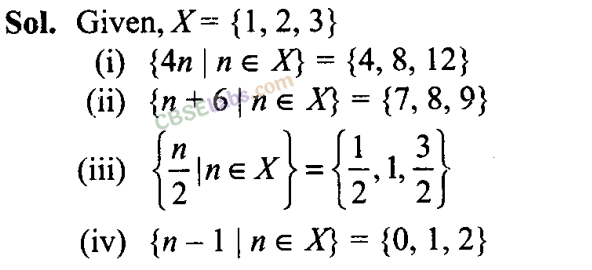Q9.If Y= {1,2,3,…, 10}, and a represents any element of Y, write the following sets, containing all the elements satisfying the given conditions.Q10. A, B and C are subsets of Universal Set If A = {2, 4, 6, 8, 12, 20}, B= {3,6,9,12,15}, C= {5,10,15,20} and U is the set of all whole numbers, draw a Venn diagram showing the relation of U, A, B and C.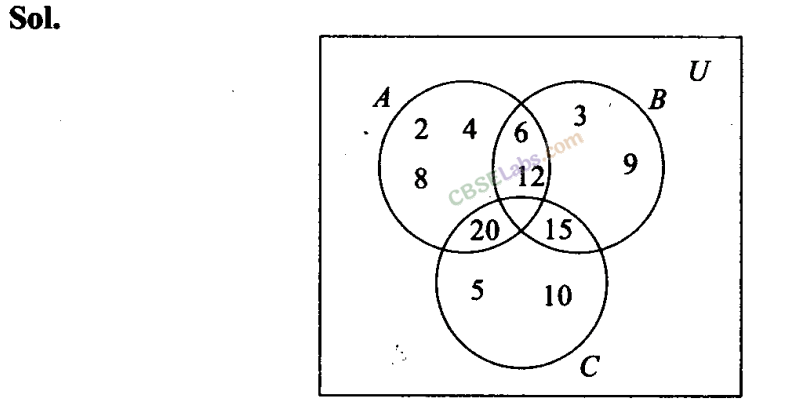Q11. Let U be the set of all boys and girls in a school, G be the set of all girls in the school, B be the set of all boys in the school, and S be the set of all students in the school who take swimming. Some, but not all, students in the school take swimming. Draw a Venn diagram showing one of the possible interrelationship among sets U, G, B and S.Q12. For all sets A, B and C, show that (A – B) ∩ (C – B) = A – (B ∪ C)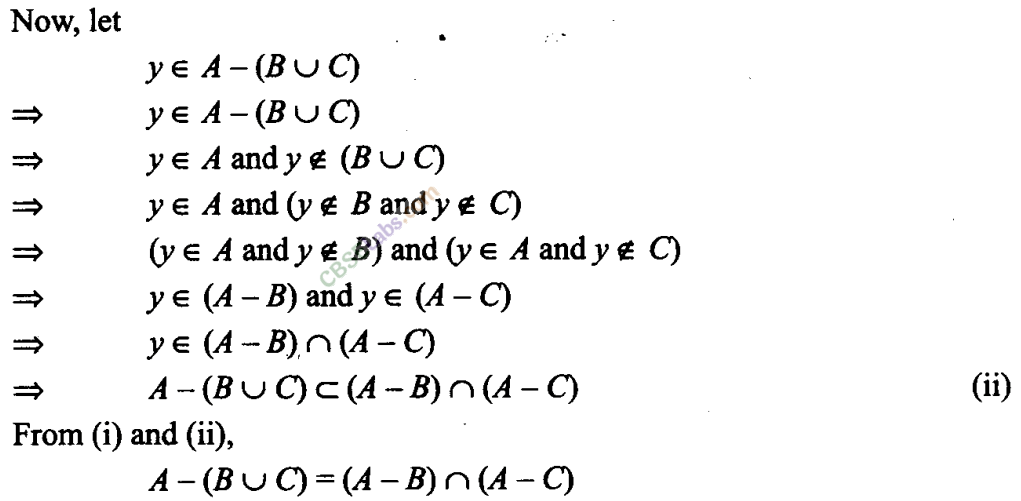Instruction for Exercises 13-17: Determine whether each of the statements in these exercises is true or false. Justify your answer.

Q13. For all sets A and B, (A – B)∪ (A∩ B) = A
Sol: True
L.H.S. = (A-B) ∪ (A∩B) = [(A-B) ∪A] ∩ [(A – B) ∪B]
= A∩ (A-B) = A= R.H.S.
Hence, given statement is true.

Q14. For all sets A, B and C, A – (B-C) = (A- B)-C
Sol: False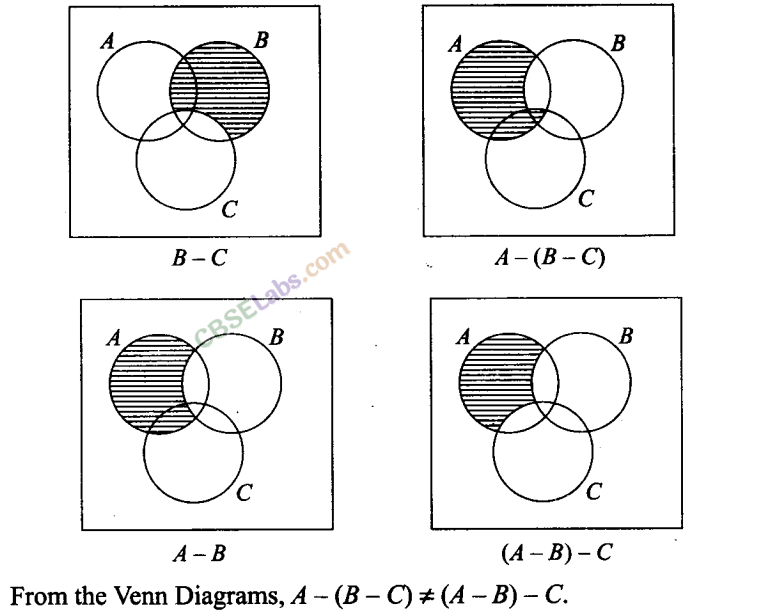Q15. For all sets A, B and C, if A ⊂ B, then  A ∩C<⊂B ∩C

Sol: True
Let          x ∈A∩C
=> x ∈ A and x∈ CQ16. For all sets A, B and C, if A⊂ B, then A ∪ C⊂ B ∪ C
Sol: TrueQ17. For all sets A, B and C, if A⊂ C and B ⊂ C,then A∪ B ⊂ CInstruction for Exercises 18-22: Using properties of sets prove the statements given in these exercises.

Q18. For all sets A and B, A ∪ (B -A) = A ∪ B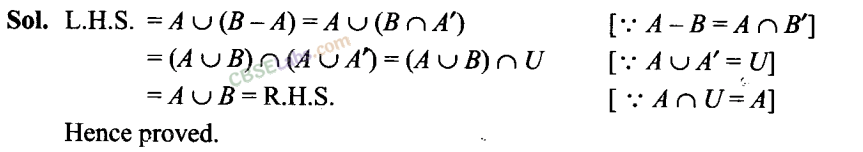Q19. For all sets A and B, A – (A – B) = A ∩ B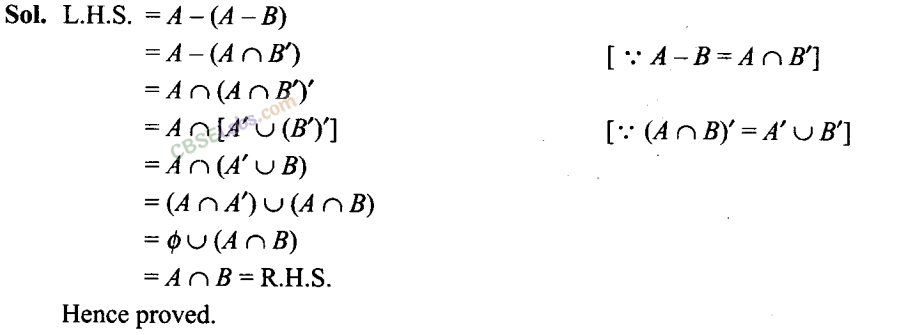Q20. For all sets A and B, A – (A ∩ B) = A – B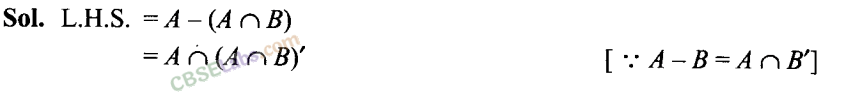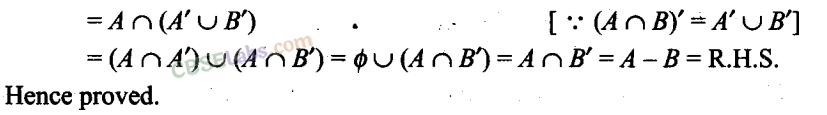Q21. For all sets A and B,(A ∪ B)- B = A-B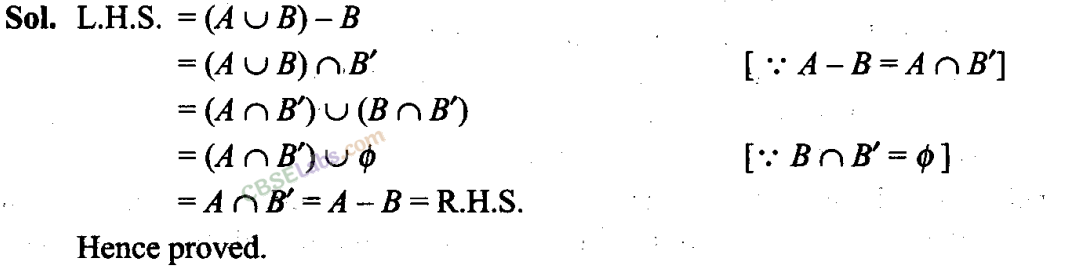Q23. Let A, B and C be sets. Then show that A ∩ (B ∪ C) = (A ∩ B)∪ (A ∩ C).From (i) and (ii), we get .                              .
A ∩ (B ∪ C) = (A ∩ B) ∪ (A ∩ C)

Q24. Out of 100 students; 15 passed in English, 12 passed in Mathematics, 8 in Science,6 in English and Mathematics, 7 in Mathematics and Science; 4 in English and Science; 4 in all the three. Find how many passed
(i) in English and Mathematics but not in Science
(ii) in Mathematics and Science but not in English
(iii) in Mathematics only
(iv) in more than one subject only
Sol. Let M be the set of students who passed in Mathematics, E be the set of students who passed in English and S be the set of students who passed in Science.

Given n (U) = 100,
n(E) = 15, n(M) = 12, n(S) = 8,
n(E ∩ M) = 6, n(M ∩S) = 7, n(E ∩ S) — 4, and n(E ∩M ∩ S) = 4,Number of students passed in English and Mathematics but not in Science = b = 2
(ii) Number of students passed in Mathematics and Science but not in English = d = 3
(iii) Number of students passed in Mathematics only = e = 3
(iv) Number of students passed in more than one subject = a + b + c + d =4+2+0+3=9

Q25. In a class of 60 students, 25 students play cricket and 20 students play tennis, and 10 students play both the games. Find the number of students who play neither.
Sol: Let C be the set of students who play cricket and T be the set of students who play tennis.
n(U) = 60, n(C) = 25, n(T) = 20, and n(C ∩ T) = 10
n(C ∪ T) = n(C) + n(T) – n(C n T) = 25 + 20 – 10 = 35

Q26. In a survey of 200 students of a school, it was found that 120 study Mathematics, 90 study Physics and 70 study Chemistry, 40 study Mathematics and Physics, 30 study Physics and Chemistry, 50 study Chemistry and Mathematics and 20 none of these subjects. Find the number of students who study all the three subjects.
Sol: Let M be the set of students who study Mathematics, P be the set of students who study E Physics and C be the set of students who study Chemistry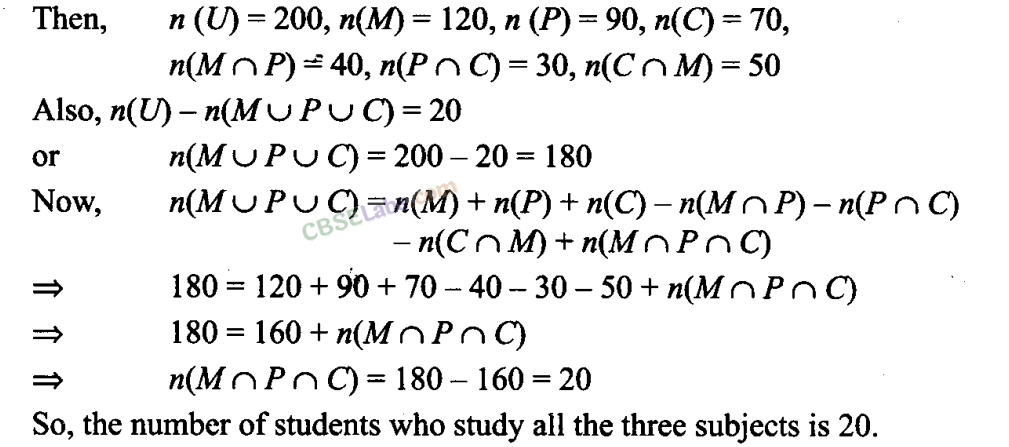Q27. In a town of 10,000 families it was found that 40% families buy newspaper A, 20% families buy newspaper B, 10% families buy newspaper C, 5% families buy A and B, 3% buy B and C and 4% buy A and C. If 2% families buy all the three newspapers. Find
(a) The number of families which buy newspaper A only.
(b) The number of families which buy none of A, B and C.
Sol:
Let A be the set of families which buy newspaper A, B be the set of families which buy newspaper B and C be the set of families which buy newspaper C. TheNumber of families which buy none of A, B and C = 10000 x (40/100)

Q28. In a group of 50 students, the number of students studying French, English, Sanskrit were found to be as follows: French = 17, English = 13, Sanskrit = 15, French and English = 09, English and Sanskrit = 4,French and Sanskrit = 5, English, French and Sanskrit = 3. Find the number of students who study
(i) French only
(ii) English only
(iii) Sanskrit only
(iv) English and Sanskrit
(v) French and  Sanskrit but not English
(vi) French and  English but not Sanskrit
(vii)     at least one  of the three languages
(viii) none of the  three languages but not French

Sol: Let F be the set of students who study French, E be the set of students who study English and S be the set of students who study Sanskrit.
Then, n{U) = 50, n(F) =17, n{E) = 13, and n{S) = 15,
n(F ∩ E) = 9, n(E ∩ S) = 4, n(F ∩ S) = 5, n(F ∩ E ∩ S) = 3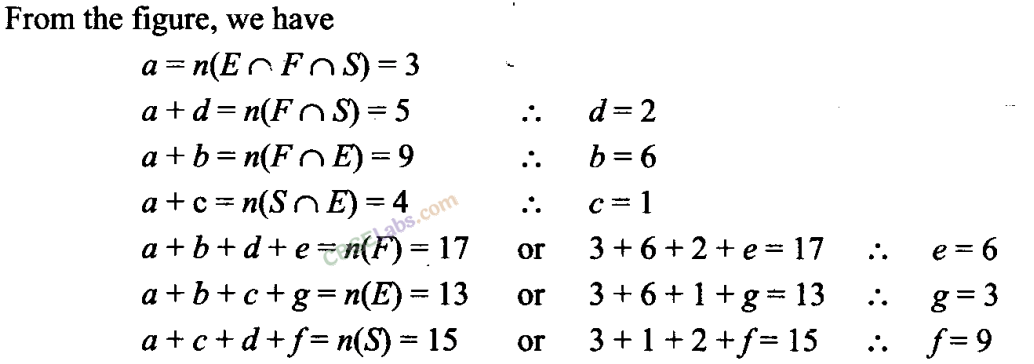(i) Number of students studying French only = e = 6
(ii) Number of students studying English only = g = 3
(iii) Number of students studying Sanskrit only =f= 9
(iv) Number of students studying English and Sanskrit but not French = c = 1
(v) Number of students studying French and Sanskrit but not English = d = 2
(vi) Number of students studying French and English but not Sanskrit = b = 6
(vii) Number of students studying at least one of the three languages = a + b + c + d + e+f+g = 30
(viii) Number of students studying none of the three languages but not French = 50-30 = 20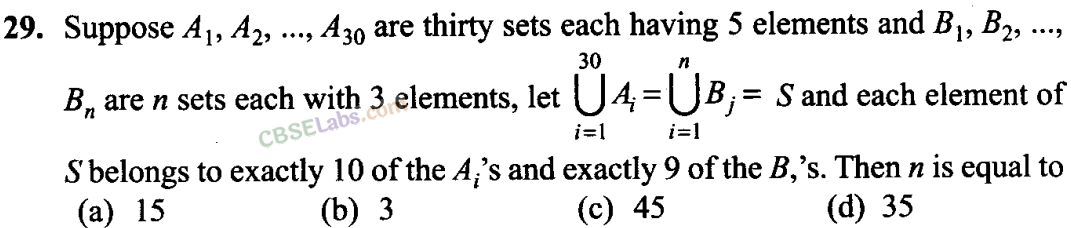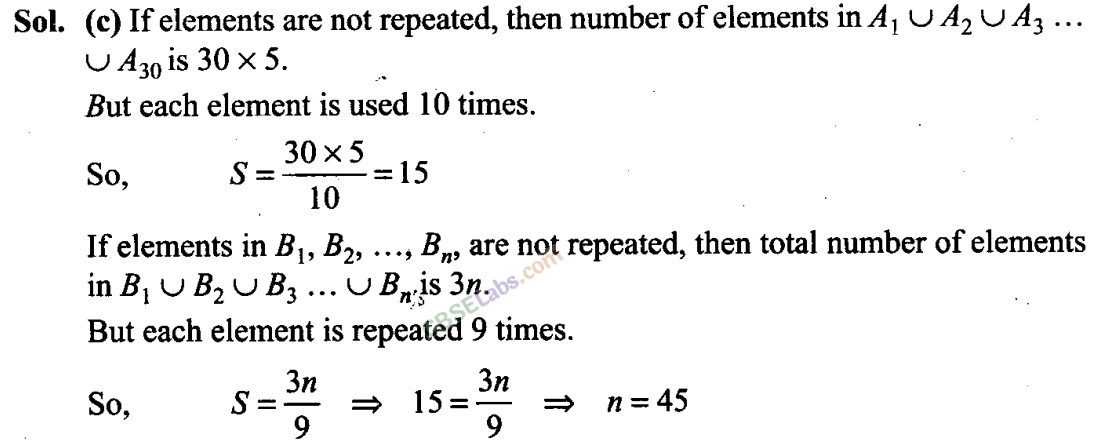Q30. Two finite sets have m and n elements. The number of subsets of the first set is 112 more than that of the second set. The values of m and n are, respectively, (a) 4,7 (b) 7,4 (c) 4,4 (d) 7, 7Q32. Let F1 be the set of parallelograms, F2 the set of rectangles, F3 the set of rhombuses, F4 the set of squares and F5 the set of trapeziums in a plane. Then F1may be equal to
(a) F2 ∩F3
(b) F3 ∩F4
(c) F2 u Fs

(d) F2 ∪ F3 ∪ F4 ∪ F1
Sol:
(d) Every rectangle, rhombus, square in a plane is a parallelogram but every trapezium is not a parallelogram.
F1 = F2 ∪ F3 ∪ F4 ∪ F1

Q33. Let S = set of points inside the square, T = the set of points inside the triangle and C = the set of points inside the circle. If the triangle and circle intersect each other and are contained in a square, then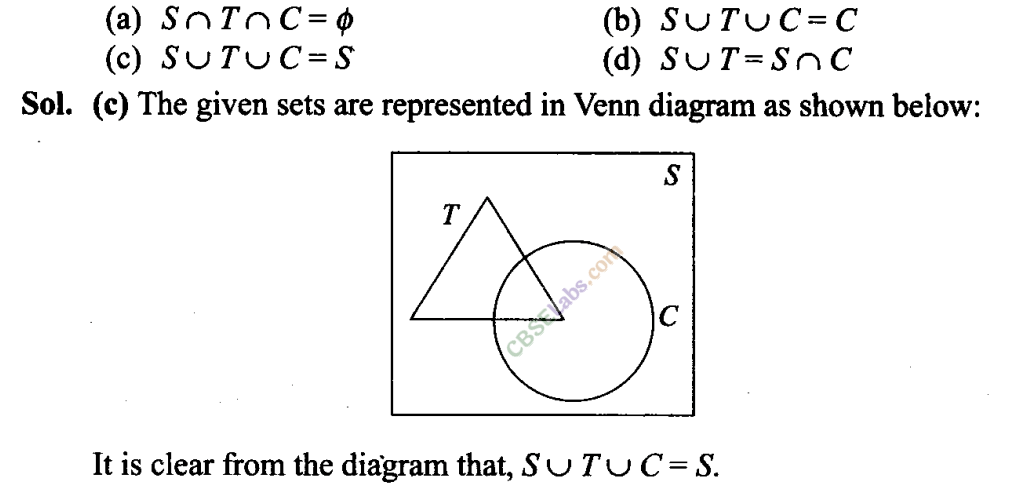Q34. Let R be set of points inside a rectangle of sides a and b (a, b> 1) with two sides along the positive direction of x-axis andy-axis. Then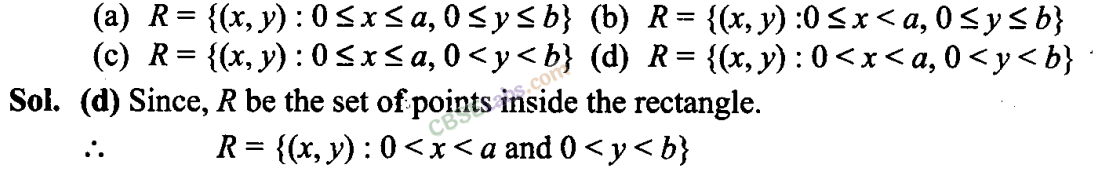Q35. In a class of 60 students, 25 students play cricket and 20 students play tennis, and 10 students play both the games. Then, the number of students who play neither is
(a) 0 (b) 25 (c) 35 (d) 45
Sol: Let C be the set of students who play cricket and T be the set of students who play tennis.
n(U) = 60, n(C) = 25, n(T) = 20, and n(C ∩ T) = 10
n(C ∪ T) = n(C) + n(T) – n(C n T) = 25 + 20 – 10 = 35

Q36. In a town of 840 persons, 450 persons read Hindi, 300 read English and 200 read both. Then the number of persons who read neither is
(a) 210 (b) 290 (c) 180 (d) 260
Sol:
(b) Let H be the set of persons who read Hindi and E be the set of persons who read English.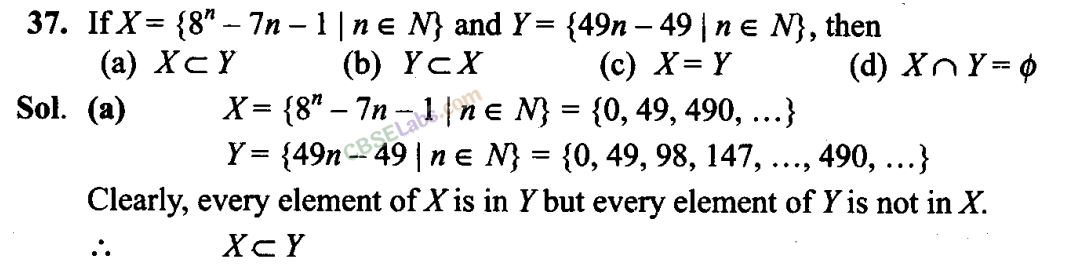We hope the NCERT Exemplar Class 11 Maths Chapter 1 Sets help you. If you have any query regarding NCERT Exemplar Class 11 Maths Chapter 1 Sets, drop a comment below and we will get back to you at the earliest.